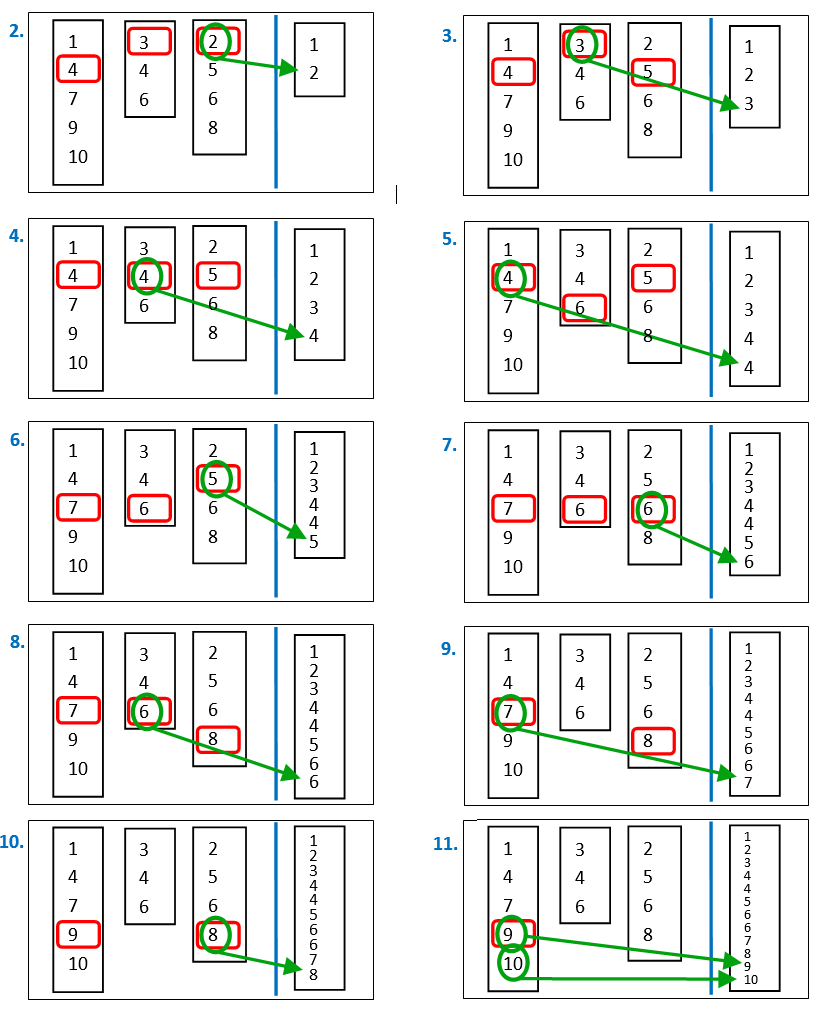﻿ 5. Detailed description of the Actions > 5.5. Standard > 5.5.2. Sort (High-Speed action) > 5.5.2.1. What are tapes? What is “Merge Sort”?

# 5.5.2.1. What are tapes? What is “Merge Sort”?

In the “Advanced Parameters” tab of the “Sort” Properties window, you will find many references to “tapes”. What are these “tapes”? The word “tape” is commonly used in the description of the sorting algorithm used inside Anatella. This algorithm is the following:

1.Create a large RAM memory buffer (whose size is given here: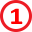) and fill this buffer with as many rows from the input table as possible.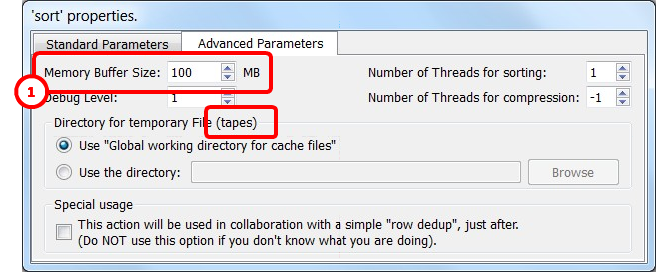2.Sort the in-memory Buffer using a classical sorting algorithm (such as Quicksort, HeapSort, etc.). Write the in-memory buffer (that is now properly sorted) on the hard drive inside a file that is typically named a “tape”. The word “tape” is used because, in the old days, the in-memory buffer was “flushed out” on real tapes such as these: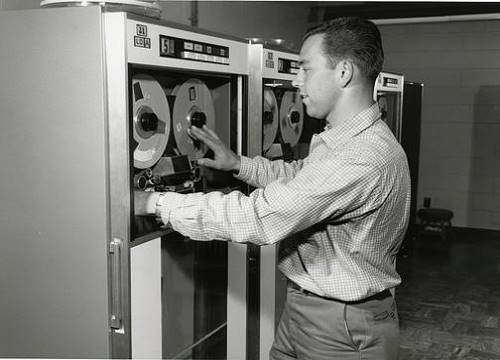3.Repeat step 1 and 2 (creating many “tape” files) up to the point that there are no more rows to read in input. At this point, the whole input table has been written into different “tape” files. Each tape is properly sorted.

We will now use an algorithm, named “Merge Sort” that reads all the different tapes and produces, as output, a table that is properly sorted. Let’s now assume (without loss of generality) that we are sorting from the smallest number to the largest number. The “Merge Sort” algorithm is the following:

4.Create a small in-memory RAM table T.

Use the first row of each of the tape files to fill-in the table T.

You’ll find on row N from table T, the first row of the tape number N.

We will now start reading all the tape files, row-by-row.

5.Search for the smallest of the rows in the table T (To remind you: we are sorting from the smallest to the largest): Let’s assume that it’s the row number X.

5.1.Send as output of the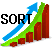Sort Action the row number X (i.e. we are adding one row to the output table).

5.2.Replace the row X from table T with the next row from the tape number X.

6.Repeat step 5 up to the point that we have read all the rows from all the tape files.

To summarize: We iterate. At each iteration, we search for the smallest row amongst all opened tape files, send it as output and replace it with the next row from the same tape file.

Here is a small illustration of the “Merge Sort” algorithm on a small example: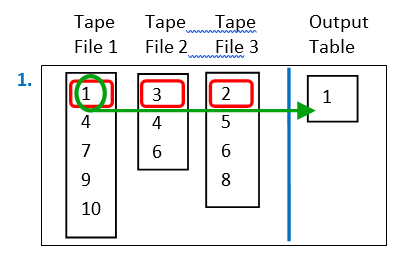Let’s assume that we have three tapes files. The first row of these three tapes file contains, respectively, the numbers 1, 3 and 2. Since we are sorting from the smallest number to the largest, we always select the smallest number and place it inside the “output” table. In the example on the left, the smallest number is “1”, so we place “1” inside the output table.

Here are the next iterations of the “Merge Sort” algorithm: